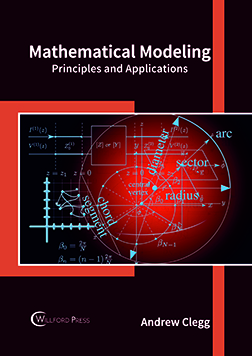BROWSE BY

#### Author

Results 1 - 1 of 1Mathematical Modeling: Principles and Applications Author : Andrew Clegg Subject : Engineering and Technology ISBN :9781682854167 Mathematical modeling is the method of developing mathematical models. This model is mainly based on mathematical language and theories and includes forms such as dynamical systems, statistical models, Read More
Results 1 - 1 of 1